# 文本相似度 (Text Similarity)

## 文本表示角度

### 统计模型

#### 文本切分

I can eat glass, it doesn’t hurt me.

• N 元语法

N-gram (N 元语法) 是一种文本表示方法，指文中连续出现的 $n$ 个词语。N-gram 模型是基于 $n-1$ 阶马尔科夫链的一种概率语言模型，可以通过前 $n-1$ 个词对第 $n$ 个词进行预测。以 南京市长江大桥 为例，N-gram 的表示如下：

一元语法（unigram）：南/京/市/长/江/大/桥


import re
from nltk.util import ngrams

s = '南京市长江大桥'
tokens = re.sub(r'\s', '', s)

list(ngrams(tokens, 1))
# [('南',), ('京',), ('市',), ('长',), ('江',), ('大',), ('桥',)]

list(ngrams(tokens, 2))
# [('南', '京'), ('京', '市'), ('市', '长'),
#  ('长', '江'), ('江', '大'), ('大', '桥')]

# [('<s>', '<s>', '南'),
#  ('<s>', '南', '京'),
#  ('南', '京', '市'),
#  ('京', '市', '长'),
#  ('市', '长', '江'),
#  ('长', '江', '大'),
#  ('江', '大', '桥'),
#  ('大', '桥', '</s>'),
#  ('桥', '</s>', '</s>')]

• 分词

s = '南京市长江大桥'

# jieba
# https://github.com/fxsjy/jieba
import jieba

list(jieba.cut(s, cut_all=False))
# ['南京市', '长江大桥']

list(jieba.cut(s, cut_all=True))
# ['南京', '南京市', '京市', '市长', '长江', '长江大桥', '大桥']

list(jieba.cut_for_search(s))
# ['南京', '京市', '南京市', '长江', '大桥', '长江大桥']

# THULAC
# https://github.com/thunlp/THULAC-Python
import thulac

thulac_ins = thulac.thulac()

thulac_ins.cut(s)
# [['南京市', 'ns'], ['长江', 'ns'], ['大桥', 'n']]

# PKUSEG
# https://github.com/lancopku/PKUSeg-python
import pkuseg

seg = pkuseg.pkuseg(postag=True)

seg.cut(s)
# [('南京市', 'ns'), ('长江', 'ns'), ('大桥', 'n')]

# HanLP
# https://github.com/hankcs/HanLP
import hanlp

tokenizer(s)
# ['南京市', '长江', '大桥']


#### 主题模型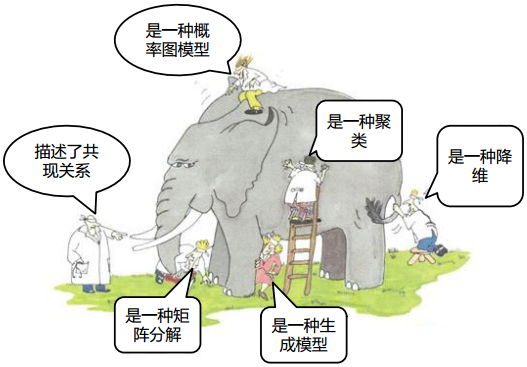• TF-IDF

TF-IDF 是 Term Frequency - Inverse Document Frequency 的缩写，即“词频-逆文本频率”。TF-IDF 可以用于评估一个字词在语料中的一篇文档中的重要程度，基本思想是如果某个字词在一篇文档中出现的频率较高，而在其他文档中出现频率较低，则认为这个字词更能够代表这篇文档。

$$w_{x, y} = tf_{x, y} \times \log \left(\dfrac{N}{df_{x}}\right)$$

14 万歌词语料 为例，通过 TF-IDF 计算周杰伦的《简单爱》中最重要的 3 个词为 ['睡着', '放开', '棒球']

• BM25

BM25 算法的全称为 Okapi BM25，是一种搜索引擎用于评估查询和文档之间相关程度的排序算法，其中 BM 是 Best Match 的缩写。

$$\operatorname{score}(D, Q)=\sum_{i=1}^{n} \operatorname{IDF}\left(q_{i}\right) \cdot \frac{f\left(q_{i}, D\right) \cdot\left(k_{1}+1\right)}{f\left(q_{i}, D\right)+k_{1} \cdot\left(1-b+b \cdot \frac{|D|}{\text { avgdl }}\right)}$$

$$\operatorname{IDF}\left(q_{i}\right)=\ln \left(\frac{N-n\left(q_{i}\right)+0.5}{n\left(q_{i}\right)+0.5}+1\right)$$

BM25 算法是对 TF-IDF 算法的优化，在词频的计算上，BM25 限制了文档 $D$ 中关键词 $q_i$ 的词频对评分的影响。为了防止词频过大，BM25 将这个值的上限设置为 $k_1 + 1$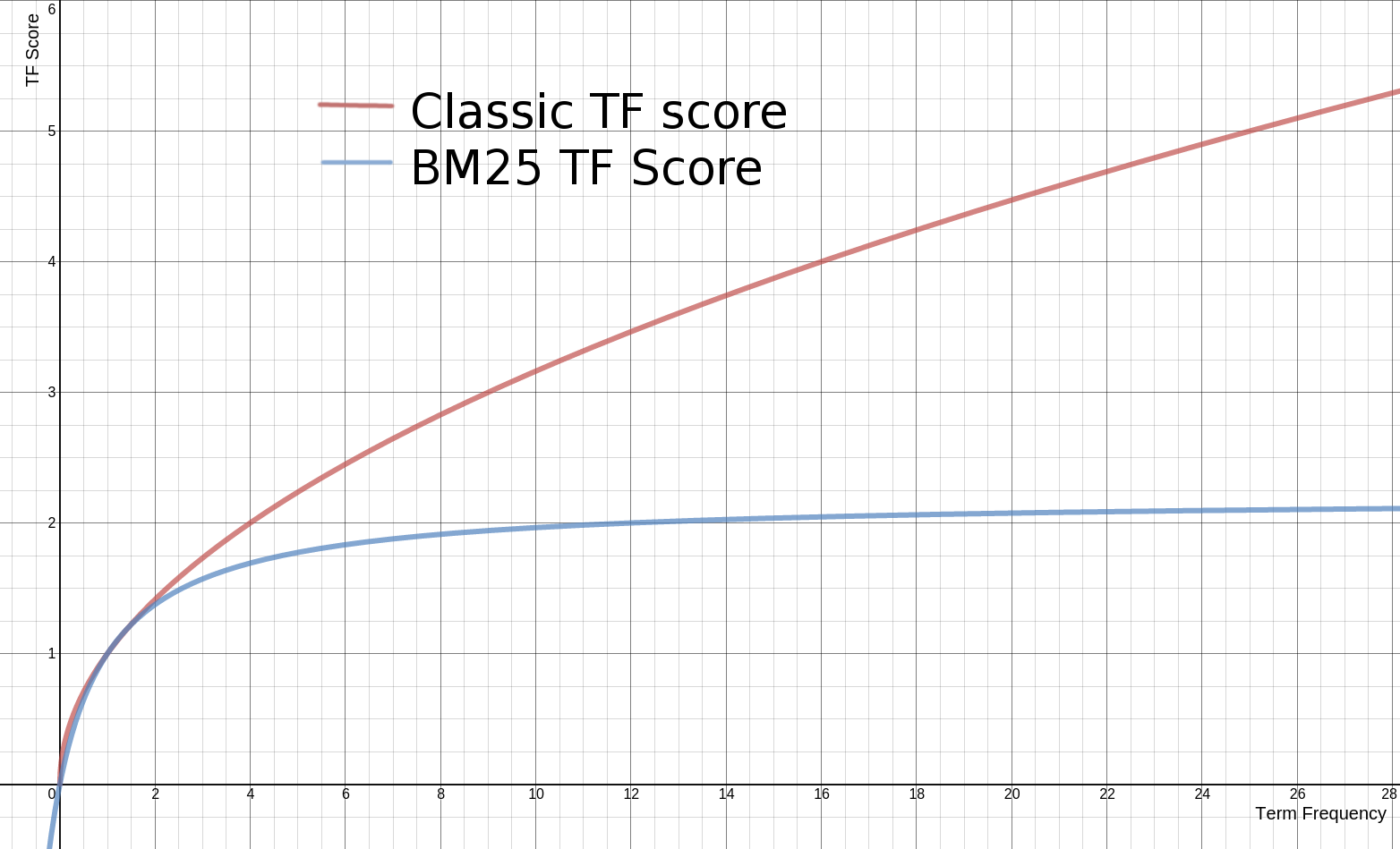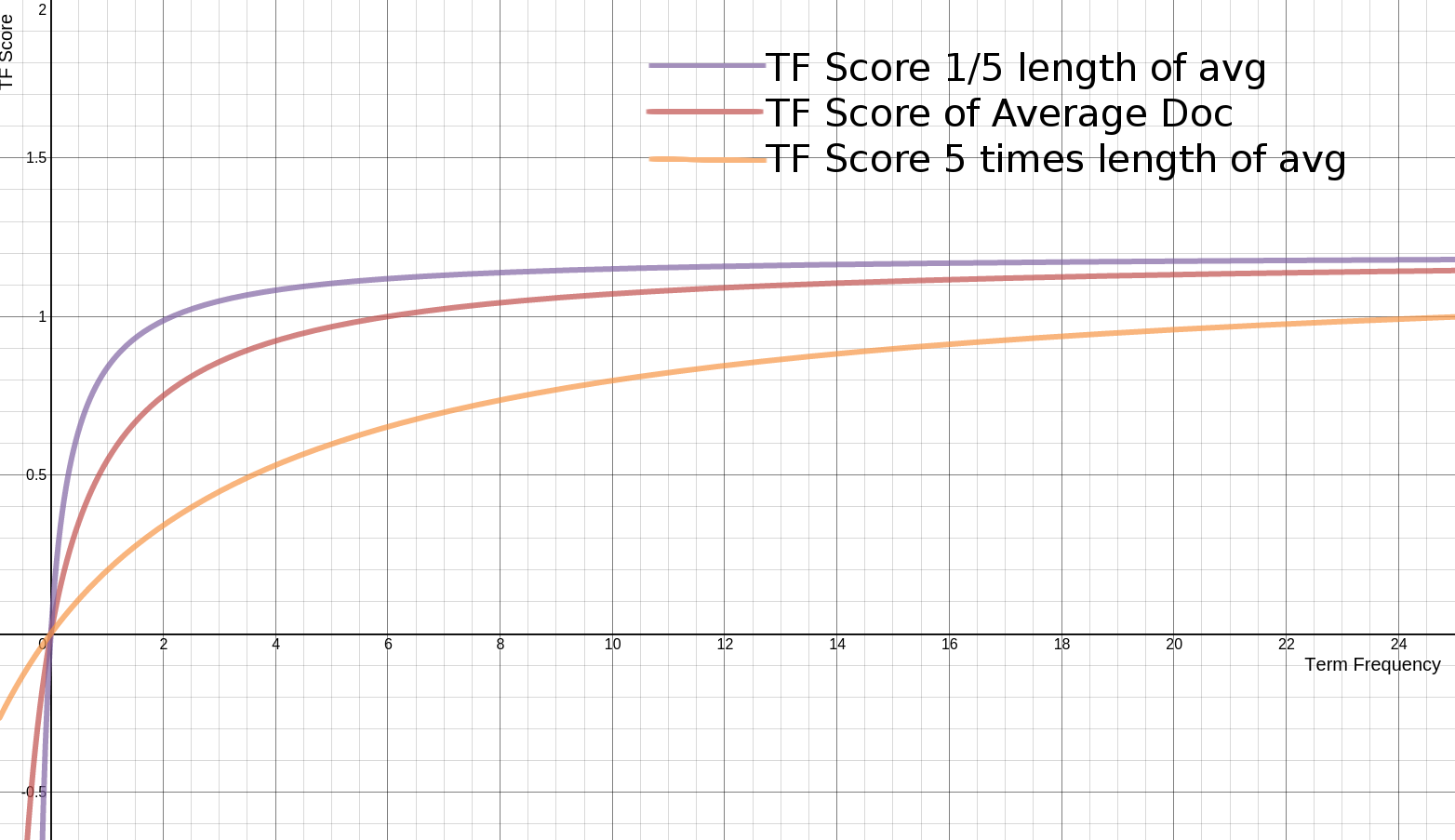• TextRank

TextRank 3 是基于 PageRank 4 算法的一种关键词提取算法。PageRank 最早是用于 Google 的网页排名，因此以公司创始人拉里·佩奇（Larry Page）的姓氏来命名。PageRank 的计算公式如下：

$$S\left(V_{i}\right)=(1-d)+d * \sum_{V_{j} \in I n\left(V_{i}\right)} \frac{1}{\left|O u t\left(V_{j}\right)\right|} S\left(V_{j}\right)$$

TextRank 由 PageRank 改进而来，计算公式如下：

$$WS \left(V_{i}\right)=(1-d)+d * \sum_{V_{j} \in In\left(V_{i}\right)} \frac{w_{j i}}{\sum_{V_{k} \in Out\left(V_{j}\right)} w_{j k}} WS \left(V_{j}\right)$$

1. 将文本进行切分得到 $S_i = \left[t_{i1}, t_{i2}, \cdots, t_{in}\right]$
2. $S_i$ 中大小为 $k$ 的滑动窗口中的词定义为共现关系，构建关键词图 $G = \left(V, E\right)$
3. 根据 TextRank 的计算公式对每个节点的值进行计算，直至收敛。
4. 对节点的 TextRank 的值进行倒叙排序，获取前 $n$ 个词作为关键词。
• LSA, PLSA, LDA & HDP

$$X = \mathbf{d}_{j} \cdot \mathbf{t}_{i}^{T} = \left[\begin{array}{c} x_{1, j} \\ \vdots \\ x_{i, j} \\ \vdots \\ x_{m, j} \end{array}\right] \cdot \left[\begin{array}{ccccc} x_{i, 1} & \ldots & x_{i, j} & \ldots & x_{i, n} \end{array}\right] = \left[\begin{array}{ccccc} x_{1,1} & \ldots & x_{1, j} & \ldots & x_{1, n} \\ \vdots & \ddots & \vdots & \ddots & \vdots \\ x_{i, 1} & \ldots & x_{i, j} & \ldots & x_{i, n} \\ \vdots & \ddots & \vdots & \ddots & \vdots \\ x_{m, 1} & \ldots & x_{m, j} & \ldots & x_{m, n} \end{array}\right]$$

$$X = U \Sigma V^{T}$$

$$\widetilde{X} =U \widetilde{\Sigma} V^{T}$$

$$\tilde{x} =\tilde{\Sigma} ^{-1} V^{T} x_{test}$$

LSA 的优点：

1. 低维空间可以刻画同义词
2. 无监督模型
3. 降维可以减少噪声，使特征更加鲁棒

LSA 的缺点：

1. 未解决多义词问题
2. 计算复杂度高，增加新文档时需要重新训练
3. 没有明确的物理解释
4. 高斯分布假设不符合文本特征（词频不为负）

PLSA 认为一篇文档可以由多个主题混合而成，而每个主题都是词上的概率分布，文章中的每个词都是由一个固定的主题生成的，如下图所示：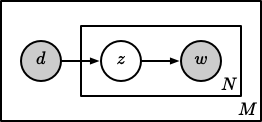$$p\left(w \mid d_{m}\right)=\sum_{z=1}^{K} p(w \mid z) p\left(z \mid d_{m}\right)=\sum_{z=1}^{K} \varphi_{z w} \theta_{m z}$$

$$p\left(\vec{w} \mid d_{m}\right)=\prod_{i=1}^{n} \sum_{z=1}^{K} p\left(w_{i} \mid z\right) p\left(z \mid d_{m}\right)=\prod_{i=1}^{n} \sum_{z=1}^{K} \varphi_{z w_{i}} \theta_{d z}$$

PLSA 可以利用 EM 算法求得局部最优解。

PLSA 优点：

1. 定义了概率模型，有明确的物理解释
2. 多项式分布假设更加符合文本特征
3. 可以通过模型选择和复杂度控制来确定主题的维度
4. 解决了同义词和多义词的问题

PLSA 缺点：

1. 随着文本和词的增加，PLSA 模型参数也随之线性增加
2. 可以生成语料中的文档的模型，但不能生成新文档的模型
3. EM 算法求解的计算量较大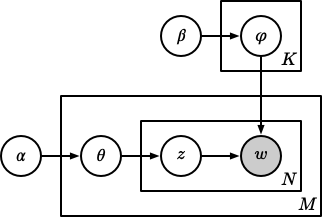LDA 的参数估计可以通过吉布斯采样实现。PLSA 和 LDA 的更多细节请参见《LDA 数学八卦》8

LDA 在使用过程中仍需要指定主题的个数，而层次狄利克雷过程（Hierarchical Dirichlet Processes, HDP）9 通过过程的构造可以自动训练出主题的个数，更多实现细节请参考论文。

LSA，PLSA，LDA 和 HDP 之间的演化关系如下图所示：#### 距离度量

$$d: X \times X \to \mathbb{R}$$

• Jaccard 系数

$$s = \dfrac{\left|X \cap Y\right|}{\left| X \cup Y \right|} = \dfrac{\left|X \cap Y\right|}{\left|X\right| + \left|Y\right| - \left|X \cap Y\right|}$$

Jaccard 系数的取值范围为：$\left[0, 1\right]$，0 表示两个集合没有重合，1 表示两个集合完全重合。

• Dice 系数

$$s = \dfrac{2 \left| X \cap Y \right|}{\left|X\right| + \left|Y\right|}$$

• Tversky 系数

$$s = \dfrac{\left| X \cap Y \right|}{\left| X \cap Y \right| + \alpha \left| X \setminus Y \right| + \beta \left| Y \setminus X \right|}$$

• Levenshtein 距离

Levenshtein 距离是 编辑距离 (Editor Distance) 的一种，指两个字串之间，由一个转成另一个所需的最少编辑操作次数。允许的编辑操作包括将一个字符替换成另一个字符，插入一个字符，删除一个字符。例如将 kitten 转成 sitting，转换过程如下：

$$\begin{equation*} \begin{split} \text{kitten} \to \text{sitten} \left(k \to s\right) \\ \text{sitten} \to \text{sittin} \left(e \to i\right) \\ \text{sittin} \to \text{sitting} \left(\ \to g\right) \end{split} \end{equation*}$$

• Jaro-Winkler 距离

$$sim = \begin{cases} 0 & \text{if} \ m = 0 \\ \dfrac{1}{3} \left(\dfrac{m}{\left|s_1\right|} + \dfrac{m}{\left|s_2\right|} + \dfrac{m-t}{m}\right) & \text{otherwise} \end{cases}$$

Jaro-Winkler 相似度给予了起始部分相同的字符串更高的分数，其定义为：

$$sim_w = sim_j + l p \left(1 - sim_j\right)$$

• 汉明距离

import textdistance as td

s1 = '南京市长江大桥'
s2 = '北京市三元桥'

td.jaccard(s1, s2)
# 0.6666666666666666

td.sorensen_dice(s1, s2)
# 0.46153846153846156

td.tversky(s1, s2)
# 0.3

td.levenshtein(s1, s2)
# 4

td.jaro(s1, s2)
# 0.6428571428571429

td.hamming(s1, s2)
# 5


### 表示学习

1. 利用表示学习方法将不定长的文本表示为定长的实值向量。
2. 计算转换后的实值向量相似度，用于表示两个文本的相似度。

## 文本词法，句法和语义角度

1. 词法，以词为对象，研究包括分词，词性和命名实体等。
2. 句法，以句子为对象，研究包括句子成分和句子结构等。
3. 语义，研究文字所表达的含义和蕴含的知识等。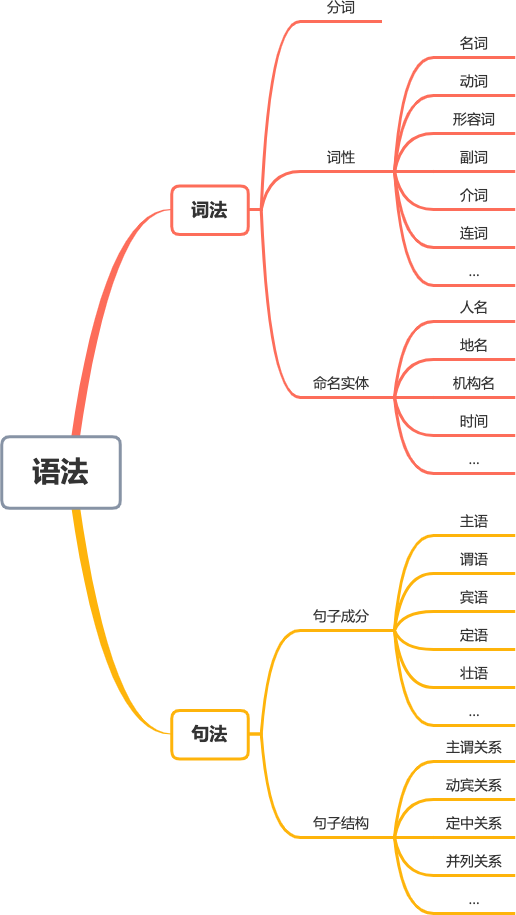### 词法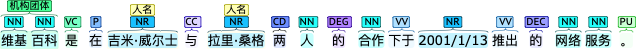### 句法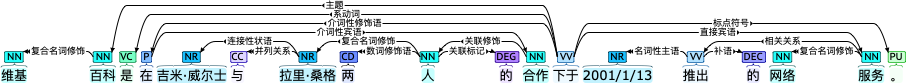### 语义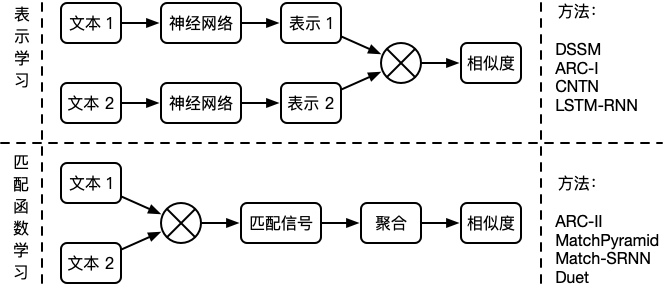## 文本长度角度

### 长文本 v.s. 长文本

1. Manning, C. D., Schütze, H., & Raghavan, P. (2008). Introduction to information retrieval. Cambridge university press. ↩︎

2. Mihalcea, R., & Tarau, P. (2004, July). Textrank: Bringing order into text. In Proceedings of the 2004 conference on empirical methods in natural language processing (pp. 404-411). ↩︎

3. Page, L., Brin, S., Motwani, R., & Winograd, T. (1999). The PageRank citation ranking: Bringing order to the web. Stanford InfoLab. ↩︎

4. Deerwester, S., Dumais, S. T., Furnas, G. W., Landauer, T. K., & Harshman, R. (1990). Indexing by latent semantic analysis. Journal of the American society for information science, 41(6), 391-407. ↩︎

5. Hofmann, T. (1999, August). Probabilistic latent semantic indexing. In Proceedings of the 22nd annual international ACM SIGIR conference on Research and development in information retrieval (pp. 50-57). ↩︎

6. Blei, D. M., Ng, A. Y., & Jordan, M. I. (2003). Latent dirichlet allocation. Journal of machine Learning research, 3(Jan), 993-1022. ↩︎

7. Rickjin(靳志辉). 2013. LDA数学八卦 ↩︎

8. Teh, Y. W., Jordan, M. I., Beal, M. J., & Blei, D. M. (2006). Hierarchical dirichlet processes. Journal of the american statistical association, 101(476), 1566-1581. ↩︎

9. 翟社平, 李兆兆, 段宏宇, 李婧, & 董迪迪. (2019). 基于词法, 句法和语义的句子相似度计算方法. 东南大学学报: 自然科学版, 49(6), 1094-1100. ↩︎

10. Zhang, K., & Shasha, D. (1989). Simple fast algorithms for the editing distance between trees and related problems. SIAM journal on computing, 18(6), 1245-1262. ↩︎

11. Meila, M., & Jordan, M. I. (2000). Learning with mixtures of trees. Journal of Machine Learning Research, 1(Oct), 1-48. ↩︎

12. Huang, P. S., He, X., Gao, J., Deng, L., Acero, A., & Heck, L. (2013, October). Learning deep structured semantic models for web search using clickthrough data. In Proceedings of the 22nd ACM international conference on Information & Knowledge Management (pp. 2333-2338). ↩︎

13. Hu, B., Lu, Z., Li, H., & Chen, Q. (2014). Convolutional neural network architectures for matching natural language sentences. In Advances in neural information processing systems (pp. 2042-2050). ↩︎

14. Qiu, X., & Huang, X. (2015, June). Convolutional neural tensor network architecture for community-based question answering. In Twenty-Fourth international joint conference on artificial intelligence↩︎

15. Palangi, H., Deng, L., Shen, Y., Gao, J., He, X., Chen, J., … & Ward, R. (2016). Deep sentence embedding using long short-term memory networks: Analysis and application to information retrieval. IEEE/ACM Transactions on Audio, Speech, and Language Processing, 24(4), 694-707. ↩︎

16. Pang, L., Lan, Y., Guo, J., Xu, J., Wan, S., & Cheng, X. (2016). Text matching as image recognition. In Proceedings of the Thirtieth AAAI Conference on Artificial Intelligence (AAAI'16). (pp. 2793–2799). ↩︎

17. Wan, S., Lan, Y., Xu, J., Guo, J., Pang, L., & Cheng, X. (2016, July). Match-SRNN: modeling the recursive matching structure with spatial RNN. In Proceedings of the Twenty-Fifth International Joint Conference on Artificial Intelligence (pp. 2922-2928). ↩︎

18. Mitra, B., Diaz, F., & Craswell, N. (2017, April). Learning to match using local and distributed representations of text for web search. In Proceedings of the 26th International Conference on World Wide Web (pp. 1291-1299). ↩︎